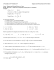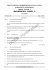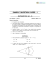# G.C.2 Wkst 3

## Transcription

G.C.2 Wkst 3
```G.C.2 STUDENT NOTES WS #3
1
Tangent Properties
As defined previously, a tangent line intersects the circle exactly once at a point called
the point of tangency. In the diagram to right, line m is a tangent line and point B is
the point of tangency. Tangent lines can possibly intersect more than one circle at a
time. For example you can have internally common tangents or externally common
tangents.
Internally Common Tangent Lines
B
A
m
Externally Common Tangent Lines
C
C
D
F
A
G
B
G
A
B
D
F
E
E
A common internal tangent intersects the segment
that joins the centers of the two circles.
A common external does not intersect the segment
that joins the centers of the two circles.
Theorem – If a line is tangent to a given circle, then the tangent line is perpendicular to
the radius at the point of tangency.
B
A
m
Proof of the Theorem -- The proof of this theorem uses an indirect approach. An
indirect proof works as follows – To prove something is true you assume that the
opposite is true and by creating a contradiction (or impossible situation) to that
assumption you actually prove that the opposite then must be true. It is kind of a
sneaky approach but in many cases it is easier to prove what something isn’t than it
is to prove what it is. Many high level proofs use an indirect approach.
So our given is tangent line m. This means that the line m intersects the circle at
point B, a point of tangency, and ALL other points on line m are exterior to the
circle. We are going to assume (the opposite of what we believe) that the angle
formed at point B is NOT a right angle (NOT 90°) and thus there exists another
segment that would be the perpendicular and represent the shortest distance from
the tangent line to point A. This creates a contradiction because for any point C it
will be in the exterior of the circle and thus a greater distance than the radius length
AB. It is impossible for AC to be shorter than AB because point C is in the exterior of
the circle and B is on the circle. This contradiction establishes that the opposite of
our assumption is true - that the point of tangency forms a right angle with the
tangent line.
A
m
B
A
m
B
C
Ultimately, when you are told that a line is tangent to a circle you gain a right angle between the tangent line
and the radius with endpoint at the point of tangency. Right angles lead to right triangles… and right triangles
lead to questions about special right triangles, trigonometry, and the Pythagorean Theorem (to name a few).
G.C.2 STUDENT NOTES WS #3
2
Example: Given that BD is a tangent line and that the radius of circle A is 5
cm and BD = 12 cm, determine ED?
A
E
x
5 cm
B
12 cm
T
D
The tangent line creates a right
triangle and so we can use the
Pythagorean Theorem.
52 + 122 = y 2
y = 13
The length of ED = 13 – 5 = 8 cm
because AE = 5 cm (radius).
Example: Given that AB is a tangent line and that AB = 4 cm, determine AT?
The tangent line creates a right
triangle and so we can use the
Pythagorean Theorem.
M
x
B
4 2 + 82 = x 2
x = 80 = 4 5 ≈ 8.94
The length of AT ≈ 8.94 cm
4 cm
A
Converse of the Theorem -- If a line is perpendicular to a radius of a circle at its
endpoint on the circle, then the line is tangent to the circle.
B
The converse is proven in a very similar method as the theorem. The converse of the
theorem gives us a way to if a line is a tangent line. We can use the Pythagorean
Theorem to test if a triangle is a right triangle or not and that helps us determine if it a
tangent line or not.
A
m
Example: Is line m a tangent line? It looks like it… not good enough….
If 32 + 42 = 62 is true then it is a right angle and line m is a tangent line.
3 cm
6 cm
m
9 + 16 ≠ 36 and so this is not a tangent line.
4 cm
Example: Is line m a tangent line to circle A?
A
If 62 + 82 = (6 + 3)2 is true then it is a right angle and line m is a tangent line.
36 + 64 ≠ 81 and so this is not a tangent line.
6 cm
8 cm
3 cm
m
Theorem – If two segments from the same exterior point are tangent to a circle,
then they are congruent to each other.
B
D
Proof of the Theorem -- The proof of this theorem is a nice review of congruent
triangles. The two tangent lines create right angles at the point of tangencies. We
also know that the two radii are congruent and that side DA is a common side.
This creates two congruent triangles ∆DBA and ∆DCA by HL. Because the two
triangles are congruent, then the sides BA and CA must be congruent.
A
C
B
D
A
C
G.C.2 STUDENT NOTES WS #3
3
C
Example -- The three segments are tangent at point B, F, and D.
If AC = 12 cm, CE = 20 cm and FE = 13 cm, determine AF?
B
If FE = 13 cm, then DE = 13 cm.
D
A
G
CE – DE = DC and so 20 – 13 = 7 cm. If DC = 7 cm, then BC = 7 cm.
F
AC – BC = AB and so 12 – 7 = 5 cm. If AB = 5 cm, then AF = 5 cm.
E
G.C.2 WORKSHEET #3
Name: ________________________________ Period ______
1. Draw the following relationships.
b) The external tangents
a) AB tangent to circle H at B.
of circle A and B.
c) In circle A, Radius AB
perpendicular to BD
B
H
1
A
A
C
2. GC is a common external tangent to circles A and B.
Explain why ∆GBD ∼ ∆GAC.
D
G
A
B
3. Solve for the missing information, given the AB is a tangent line to circle C.
a)
b)
c)
B
d)
A
10 cm
C
45°
A
C
C
55°
6 cm
F
15 cm
C
8 cm
D
8 cm
B
B
e)
f)
C
5 cm
8 cm
h)
B
A
12 cm
C
C
C
30°
A
CB = __________ (E)
A
B
G
2 cm
AB = __________
FA = __________
g)
D
B
9 cm
B
A
CB = __________
AC = __________
CB = __________
A
4 cm
CB = __________ (2 dec.)
B
10 3 cm
A
CB = __________
G.C.2 WORKSHEET #3
2
4. Determine if the AB is a tangent line or not.
a)
b)
c)
d)
A
8 3 cm
B
A
B
17 cm
C
15 cm
9 cm
15 cm
C
C
20 cm
12 cm
A
Yes or No
C
8 cm
B
25 cm
B
8 cm
F
A
52 cm
Yes or No
Yes or No
Yes or No
5. Given that AB is tangent to circle C and EA = 9 cm and AB = 15 cm,
determine CB. (Hint: Label the two radii with x)
C
E
B
15 cm
6. Solve for x ( AB and AD are tangent lines)
a)
b)
9 cm
A
c)
D
D
12 cm
C
C
D
45°
B
C
77 cm
A
x
B
x2 - 3
A
5x - 3
13 cm
B
A
x = ____________
x = ____________
x = ____________
7. Solve for the missing information (Lines that appear to be tangent are tangent.)
a) AC = 18 cm, CE = 30 cm & AF = 10 cm
b) Perimeter = 40 cm, AC = 15 cm, AF = 8.5 cm
C
C
D
B
B
D
G
G
A
A
F
F
E
FE = _____________
E
FE = _____________
xo^r,
G.C.z WORKSHEET #3
W
period
1. Draw the following relationships.
d 7E tangent to circle H at B.
2. de
is a common
Explain why AGBD
-
b) The external tangents
of circle A and B.
externaltangent to circles A and
perpendicular to ED
B.
AGAC.
3. Solve for the missing information, given the AB is a tangent line to circle C.
b)
c)
cm
@@
Wq
-tang-'=
Y>
cB
= € C't*
AC=
cB
T
h\
= b1o
cr,^n
FA=
e)
Ar'+ 1*= l3z
L-Y z{= Ib\
&g--.1ln-*
b
->=.!
AT
eo#'(wtL
6t_>
ss= lL
cM-
trrtr
X=ff-
X.=ZJt-
CB=
(E)
cB
lB
= /-fficn
(2 dec.)
CB=
G.C.2 WORKSHEET #3
4. Determine if the
a)
7E ita tangent line or not.
b)
Yes
or(No)
\1
Yes
or No
/Yes)or
Yes
\Z
5. Given that VE is tangent to circle C and EA = 9 cm and AB = 15 cm,
determine CB. (Hint: Label the two radiiwith x)
Xt+ @s-= k+<)y- +Lzf : X-+kY+81
,vrf =- tF f +f l
rt,{ -- LtY *2 Y:lt c/^ \
6. Solve for x AB and AD aretangent lin"ri-(
f*t = t7
i: lt"
Y9-v
C
a
B
x= &tlcw
x=
7. Solve for the missing information (Lines that appear to be tangent are tangent.)
a) AC = 18 cm, CE = 30cm & AF = L0 cm
b) Perimeter = 40 cm, AC = L5 cm, AF = 8.5 cm
c
*1.
FE
= /2'
Cvtvt
FE=
or
No
```

### Name: __________________________________________________________________ Period: ___________________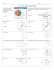### Math 083 Sample Exam 3 A Given: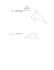### The great angle chase: Student worksheet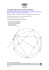### square, circle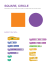### AP Calculus AB - Worksheet 19